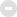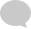##用戶名 Email 自動登錄 找回密碼 密碼 會員申請加入
 搜索
﻿

 (B0)香港巴士車務及車廂設備 (B1)香港巴士廣告消息/廣告車行踪 (B2)香港巴士討論 [熱門] [精華] (B3)巴士攝影作品貼圖區 [熱門] [精華] (B3i)即拍即貼 -手機相&翻拍Mon相 (B4)兩岸三地巴士討論 [精華] (B5)外地巴士討論 [精華] Advertisement (B6)旅遊巴士及過境巴士 [精華] (B7)巴士特別所見 (B11)巴士精華區 (B22)巴士迷吹水區 (V)私家車,商用車,政府及特種車輛 [精華] (A6)相片及短片分享/攝影技術 (A10)香港地方討論 [精華] (A11)消費著數及飲食資訊 (A16)建築物機電裝置及設備 (A19)問路專區 (N)其他討論題目 (F1)交通路線建議 (C2)航空 [精華] (C3)海上交通及船隻 [精華] (D1)公共交通有關商品 [精華] (Y)hkitalk.net會員福利部 (Z)站務資源中心 (R1)香港鐵路 [精華] (R2)香港電車 [精華] (R3)港外鐵路 [精華] (O1)omsi討論區 (O2)omsi下載區 (O3)omsi教學及求助區 (M1)小型巴士綜合討論 (M2)小型巴士多媒體分享區 (M3)香港小型巴士字軌表hkitalk.net 香港交通資訊網»論壇 Notice

#### 熱帖## Notice